# Colleges with the lowest SAT scores in North Dakota

Top 5 colleges in North Dakota with the lowest SAT scores
Looking for the colleges with the lowest SAT scores in North Dakota? Well you're in luck! We've compiled a national college database and have created a list of the top 5 universities with the lowest SAT scores in North Dakota below. If you are not a good test taker or worried about your test scores, this list is for you. These are the schools whose applicants had the lowest average SAT scores in North Dakota, which means that you can get into these colleges with a lower SAT score. We also include each college's ACT scores and acceptance rate so that you can see where you would have the easiest time getting in. Read on to find out more.

## Valley City State University SAT scores

The average SAT score for Valley City State University is 1024.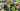The average SAT score of 1024 breaks down into:

• SAT math: 520

The average ACT score for Valley City State University is 21 and their acceptance rate is 73.7%.

## Minot State University SAT scores

The average SAT score for Minot State University is 1030.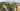The average SAT score of 1030 breaks down into:

• SAT math: 520

The average ACT score for Minot State University is 22 and their acceptance rate is 50.1%.

## Mayville State University SAT scores

The average SAT score for Mayville State University is 1040.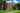The average SAT score of 1040 breaks down into:

• SAT math: 520

The average ACT score for Mayville State University is 20 and their acceptance rate is 57.1%.

## University of North Dakota SAT scores

The average SAT score for University of North Dakota is 1110.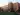The average SAT score of 1110 breaks down into:

• SAT math: 570

The average ACT score for University of North Dakota is 23 and their acceptance rate is 82.2%.

## North Dakota State University SAT scores

The average SAT score for North Dakota State University is 1170.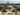The average SAT score of 1170 breaks down into:

• SAT math: 590